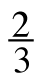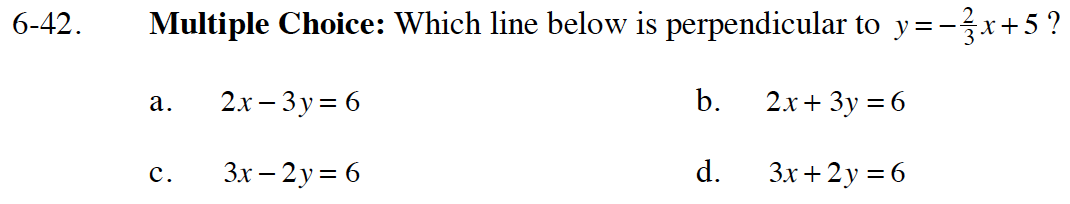Home > GB8I > Chapter cc46 > Lesson cc46.1.4 > Problem6-42

6-42.
1. Multiple Choice: Which line below is perpendicular to y = −x + 5? Homework Help ✎

1. 2x − 3y = 6

2. 2x + 3y = 6

3. 3x − 2y = 6

4. 3x + 2y = 6Rearrange the equations into Slope-Intercept form. Remember that parallel lines have equal slopes.